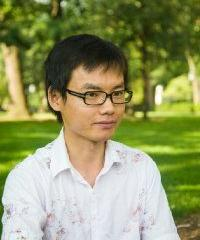Homogenization Theory, Random Fluctuations and Inverse Problems
Speaker
A/Prof. Wen-Jia Jing
Yau Mathematical Sciences Center, Tsinghua University
Abstract

Partial differential equations with oscillatory coefficients arise in many applications, such as the modeling of composite materials and flame propagations. The fine scale oscillations of the physical media are often unknown and, hence, modeled as random. The theory of stochastic homogenization amounts to exploring the self-averaging mechanism of the PDEs, and it leads to mean field approximations of large scale behaviors of the solution. The theory of random fluctuations aims at studying the difference between the solution and its homogenization limit, and it leads to higher order, and very often Gaussian, corrections to the approximation. In this talk, I will present some results on stochastic homogenization, fluctuation theory for elliptic equations with random potentials and some related numerical analysis and applications in inverse problems.